﻿

Forest fires prediction using machine learning

# Predict forest fires using artificial intelligence

In this example, we develop a classification method for predicting forest fires.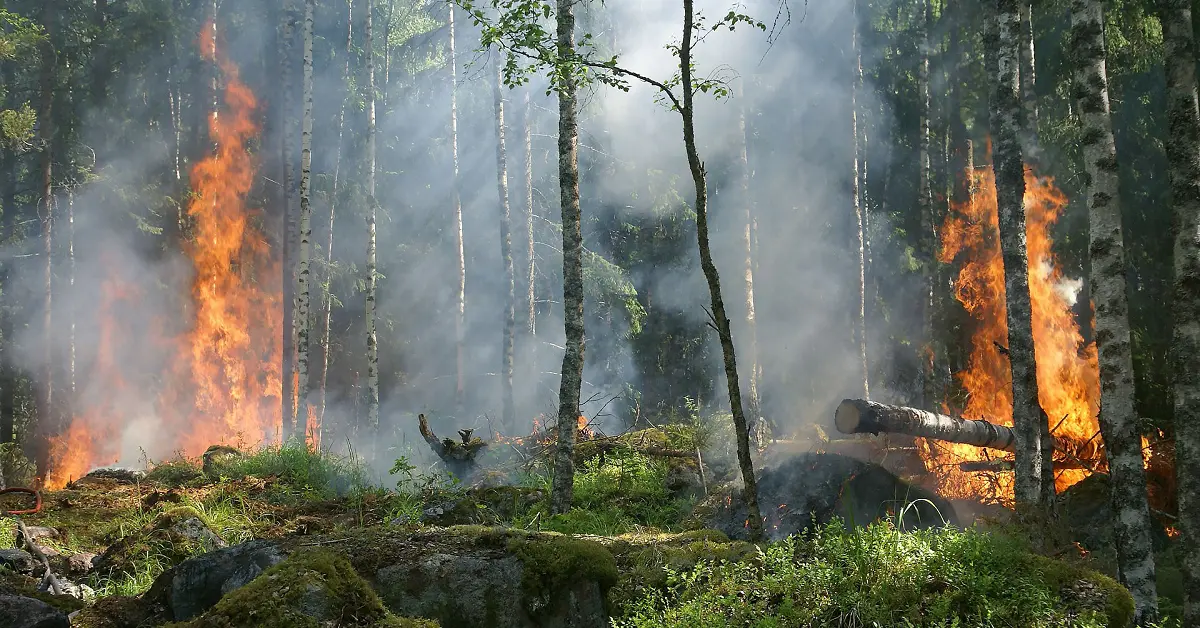### Contents:

This example is solved with Neural Designer. To follow it step by step, you can use the free trial.

## 1. Application type

This is a classification project, since the variable to be predicted is binary (fire or not fire).

The goal here is to model the probability of a fire occurring according to the day, month, certain meteorological variables, and a series of indices developed by the FWI system.

## 2. Data set

The data set comprises a data matrix in which columns represent variables, and rows represent instances.

The data file forestfires.csv contains the information for creating the model. Here, the number of variables is 9, and the number of instances is 515.

This data set contains the following variables whose contain data measured in the northeast region of Portugal:

• month
• FFMC: index FWY system: Fine Fuel Moisture Code.
• DMC: index FWY system: Duff Moisture Code.
• DC: index FWY system: Drought Code.
• ISI: index FWY system: Initial Spread Index.
• temp: temperature in Celsius degrees
• RH: relative humidity in %.
• wind: wind speed in km/h.
• class: (target) 1:fire 0:not fire.

The total number of instances is 515. They are divided into training, generalization, and testing subsets. The number of training instances is 333 (80%), the number of selection instances is 45 (10%), and the number of testing instances is 45 (10%).

Once the data set has been set, we can perform a few related analytics. First, we check the provided information and ensure that the data has good quality.

We can calculate the data statistics and draw a table with the minimums, maximums, means, and standard deviations of all variables in the data set. The following table depicts the values.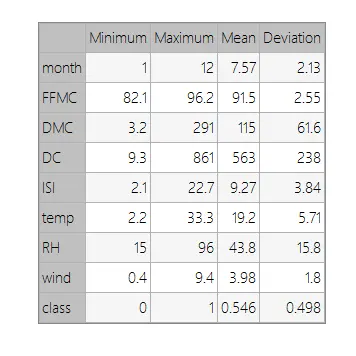Also, we can calculate the distributions for all variables. The following figure shows a pie chart with the proportion of fire (positives) and not fire (negatives) following our dataset.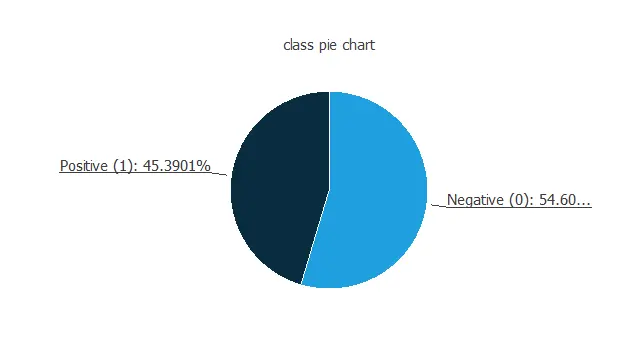As we can see, the number of fire cases is 45.4% of the samples, and not fire represents approximately 54.6% of the pieces.

Finally, the inputs-targets correlations might indicate to us what factors most influence fires.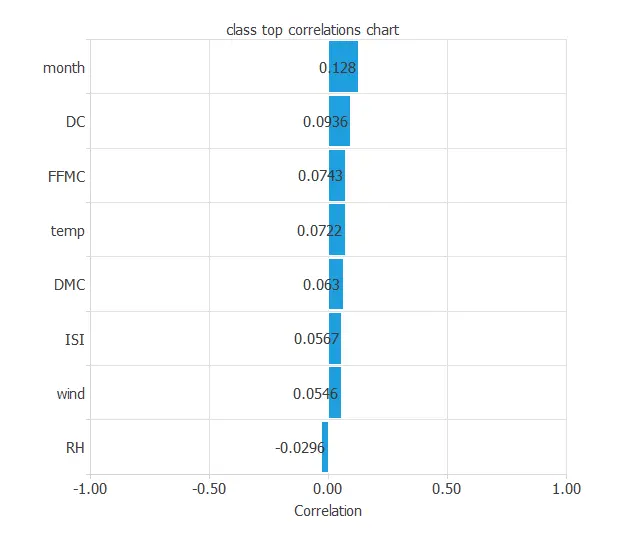Here, the different variables are little correlated. Indeed, forest fire depends on many factors at the same time.

## 3. Neural network

The second step is to set a neural network to represent the classification function. For this class of applications, the neural network is composed of:

• Scaling layer.
• Perceptron layers.
• Probabilistic layer.

The scaling layer contains the statistics on the inputs calculated from the data file and the method for scaling the input variables. Here the minimum and maximum methods have been set. Nevertheless, the mean and standard deviation methods would produce very similar results.

The number of perceptron layers is 1. This perceptron layer has 8 inputs and 8 neurons.

Finally, we will set the binary probabilistic method for the probabilistic layer as we want the predicted target variable to be binary.

The following picture shows a graph of the neural network for this example.The yellow circles represent scaling neurons, the blue circles perceptron neurons, and the red circles probabilistic neurons. The number of inputs is 8, and the number of outputs is 1.

## 4. Training strategy

The procedure used to carry out the learning process is called a training strategy. The training strategy is applied to the neural network to obtain the best possible performance. The type of training is determined by how the adjustment of the parameters in the neural network takes place. This process is composed of two terms:

• A loss index.
• An optimization algorithm.

The loss index that we use is the weighted squared error with L2 regularization. This is the default loss index for binary classification applications.

The learning problem is finding a neural network that minimizes the loss index. That is, a neural network that fits the data set (error term) and does not oscillate (regularization term).

The optimization algorithm that we use is the quasi-Newton method. This is also the standard optimization algorithm for this type of problem.

The following chart shows how the error decreases with the iterations during the training process.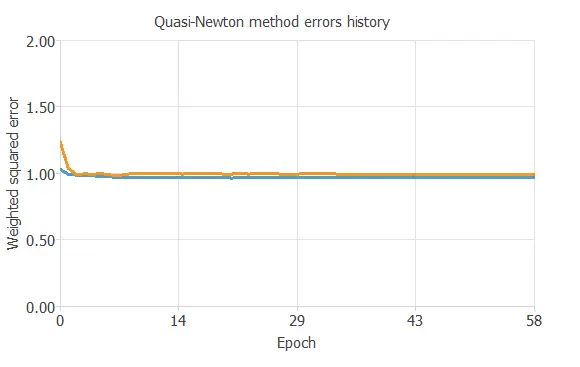The final training and selection errors are training error = 0.966 WSE and selection error = 0.933 WSE, respectively.

## 5. Model selection

The objective of model selection is to find the network architecture with the best generalization properties, that is, that which minimizes the error on the selected instances of the data set.

More specifically, we want to find a neural network with a selection error of less than 0.933 WSE, which is the value that we have achieved so far.

Order selection algorithms train several network architectures with a different number of neurons and select that with the smallest selection error.

The incremental order method starts with a small number of neurons and increases the complexity at each iteration.

## 6. Testing analysis

The last step is to test the generalization performance of the trained neural network.

The objective of the testing analysis is to validate the generalization performance of the trained neural network. To validate a classification technique, we need to compare the values provided by this technique to the observed values. We can use the ROC curve as it is the standard testing method for binary classification projects.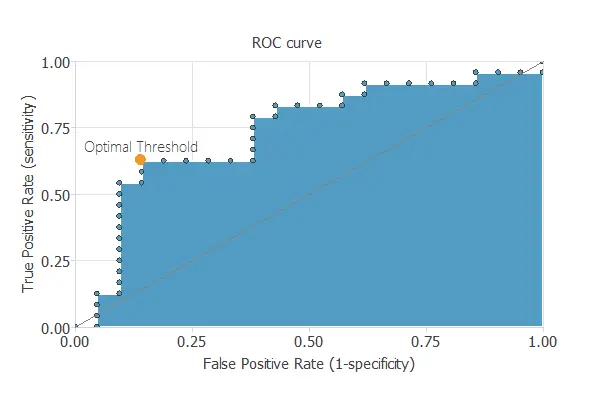In the confusion matrix, the rows represent the target classes and the columns the output classes for the testing target data set. The diagonal cells in each table show the number of correctly classified cases, and the off-diagonal cells show the misclassified instances.

The following table shows the confusion elements for this application. The following table contains the elements of the confusion matrix.

Predicted positive Predicted negative
Real positive 13 (28.9%) 8 (18.7%)
Real negative 7 (15.6%) 17 (37.8%)

As we can see, the number of instances that the model can correctly predict is 30 (66.7%), while it misclassifies 15 (33.3%).

The next list depicts the binary classification tests for this application:

• Classification accuracy: 66.7% (ratio of correctly classified samples).
• Error rate: 33.3% (ratio of misclassified samples).
• Sensitivity: 61.9% (percentage of actual positive classified as positive).
• Specificity: 70.8% (percentage of actual negative classified as negative).

## 7. Model deployment

The neural network is now ready to predict outputs for inputs that it has never seen.

Below, a specific prediction having determined values for the model’s input variables is shown.

• month: 6 (June)
• FFMC: 75.12
• DMC: 94.26
• DC: 462.3
• ISI: 7.6
• temperature: 15.8 ºC
• RH: 36.0 %
• wind: 3.3 km/h
• Fire probability: 74 %

The model predicts that the previous values correspond to a probability of fire is about 74%.

We can now use Response Optimization. The objective of the response optimization algorithm is to exploit the mathematical model to look for optimal operating conditions.
Indeed, the predictive model allows us to simulate different operating scenarios and adjust the control variables to improve efficiency.

An example is to minimize fire probability in a fixed month.

The next table resumes the conditions for this problem.

Variable name Condition
Month Equal to 9
FFMC None
DMC None
ISI None
Temperature None
RH None
Wind speed None
Fire probability Minimize

The next list shows the optimum values for previous conditions.

• month: 9 (September)
• FFMC: 79.5756.
• DMC: 71.5245.
• DC: 730.775.
• ISI: 22.943.
• temperature: 7.719 ºC.
• RH: 38.0269 %.
• wind: 0.650214 km/h.
• Fire probability: 0.1 %

The mathematical expression, represented by the neural network, which can be exported to any specific software, is written below.

scaled_month = month*(1+1)/(12-(1))-1*(1+1)/(12-1)-1;
scaled_FFMC = FFMC*(1+1)/(96.19999695-(82.09999847))-82.09999847*(1+1)/(96.19999695-82.09999847)-1;
scaled_DMC = DMC*(1+1)/(291.2999878-(3.200000048))-3.200000048*(1+1)/(291.2999878-3.200000048)-1;
scaled_DC = DC*(1+1)/(860.5999756-(9.300000191))-9.300000191*(1+1)/(860.5999756-9.300000191)-1;
scaled_ISI = ISI*(1+1)/(22.70000076-(2.099999905))-2.099999905*(1+1)/(22.70000076-2.099999905)-1;
scaled_temp = temp*(1+1)/(33.29999924-(2.200000048))-2.200000048*(1+1)/(33.29999924-2.200000048)-1;
scaled_RH = RH*(1+1)/(96-(15))-15*(1+1)/(96-15)-1;
scaled_wind = wind*(1+1)/(9.399999619-(0.400000006))-0.400000006*(1+1)/(9.399999619-0.400000006)-1;

perceptron_layer_0_output_0 = logistic[ 0.595337 + (scaled_month*0.365295)+ (scaled_FFMC*-0.421814)+ (scaled_DMC*0.666443)+ (scaled_DC*0.126282)+ (scaled_ISI*-0.1427)+ (scaled_temp*0.479919)+ (scaled_RH*-0.277588)+ (scaled_wind*-0.0090332) ];
perceptron_layer_0_output_1 = logistic[ 0.745605 + (scaled_month*0.215881)+ (scaled_FFMC*0.39386)+ (scaled_DMC*0.468689)+ (scaled_DC*-0.083252)+ (scaled_ISI*-0.942017)+ (scaled_temp*0.669189)+ (scaled_RH*-0.74292)+ (scaled_wind*0.649841) ];
perceptron_layer_0_output_2 = logistic[ 0.490295 + (scaled_month*-0.260254)+ (scaled_FFMC*-0.546326)+ (scaled_DMC*-0.00305176)+ (scaled_DC*0.404602)+ (scaled_ISI*0.790894)+ (scaled_temp*0.639038)+ (scaled_RH*-0.43158)+ (scaled_wind*-0.126587) ];
perceptron_layer_0_output_3 = logistic[ -0.0189209 + (scaled_month*-0.864746)+ (scaled_FFMC*-0.548584)+ (scaled_DMC*-0.697632)+ (scaled_DC*-0.962402)+ (scaled_ISI*-0.0921631)+ (scaled_temp*0.247986)+ (scaled_RH*-0.161255)+ (scaled_wind*-0.158508) ];
perceptron_layer_0_output_4 = logistic[ 0.399719 + (scaled_month*0.683105)+ (scaled_FFMC*-0.915894)+ (scaled_DMC*-0.323242)+ (scaled_DC*-0.207825)+ (scaled_ISI*0.925049)+ (scaled_temp*0.611633)+ (scaled_RH*0.728943)+ (scaled_wind*0.547729) ];
perceptron_layer_0_output_5 = logistic[ 0.274475 + (scaled_month*0.472839)+ (scaled_FFMC*-0.127686)+ (scaled_DMC*-0.808655)+ (scaled_DC*0.556091)+ (scaled_ISI*-0.12561)+ (scaled_temp*0.547974)+ (scaled_RH*-0.957092)+ (scaled_wind*-0.192749) ];
perceptron_layer_0_output_6 = logistic[ 0.846924 + (scaled_month*0.379395)+ (scaled_FFMC*0.230347)+ (scaled_DMC*0.486023)+ (scaled_DC*-0.238708)+ (scaled_ISI*0.405518)+ (scaled_temp*0.272766)+ (scaled_RH*0.641235)+ (scaled_wind*0.514526) ];
perceptron_layer_0_output_7 = logistic[ 0.772766 + (scaled_month*0.289612)+ (scaled_FFMC*0.786621)+ (scaled_DMC*0.808289)+ (scaled_DC*0.266663)+ (scaled_ISI*0.136658)+ (scaled_temp*0.344666)+ (scaled_RH*-0.394348)+ (scaled_wind*-0.848816) ];

probabilistic_layer_combinations_0 = 0.578857 +0.953979*perceptron_layer_0_output_0 +0.302429*perceptron_layer_0_output_1 +0.491089*perceptron_layer_0_output_2 -0.742981*perceptron_layer_0_output_3 -0.139282*perceptron_layer_0_output_4 -0.347168*perceptron_layer_0_output_5 -0.456177*perceptron_layer_0_output_6 +0.837708*perceptron_layer_0_output_7

class = 1.0/(1.0 + exp(-probabilistic_layer_combinations_0);

logistic(x){
return 1/(1+exp(-x))
}



With the previous algorithm created (the mathematical expression), we can predict the fire risk and generate a fire map risk. The following images show an example of such a map:The image above shows the Portugal forest fire risk.

## References

• The data for this problem has been taken from the UCI Machine Learning Repository.
• [Cortez and Morais, 2007] P. Cortez and A. Morais. A Data Mining Approach to Predict Forest Fires using Meteorological Data. In J. Neves, M. F. Santos and J. Machado Eds., New Trends in Artificial Intelligence, Proceedings of the 13th EPIA 2007 – Portuguese Conference on Artificial Intelligence, December, Guimares, Portugal, pp. 512-523, 2007. APPIA, ISBN-13 978-989-95618-0-9. Web Link.
• Portugal fire risk map image Web Link.